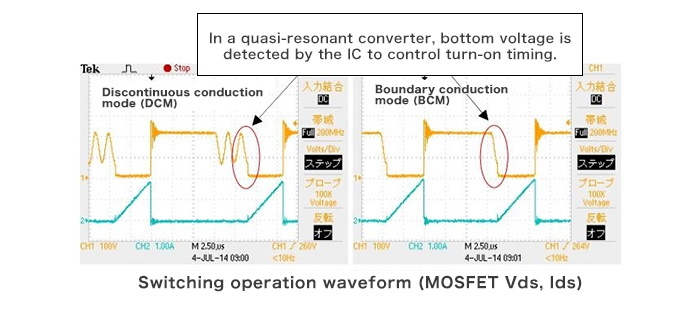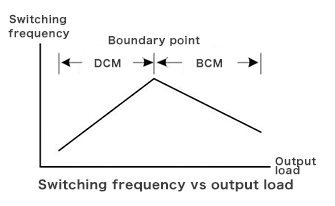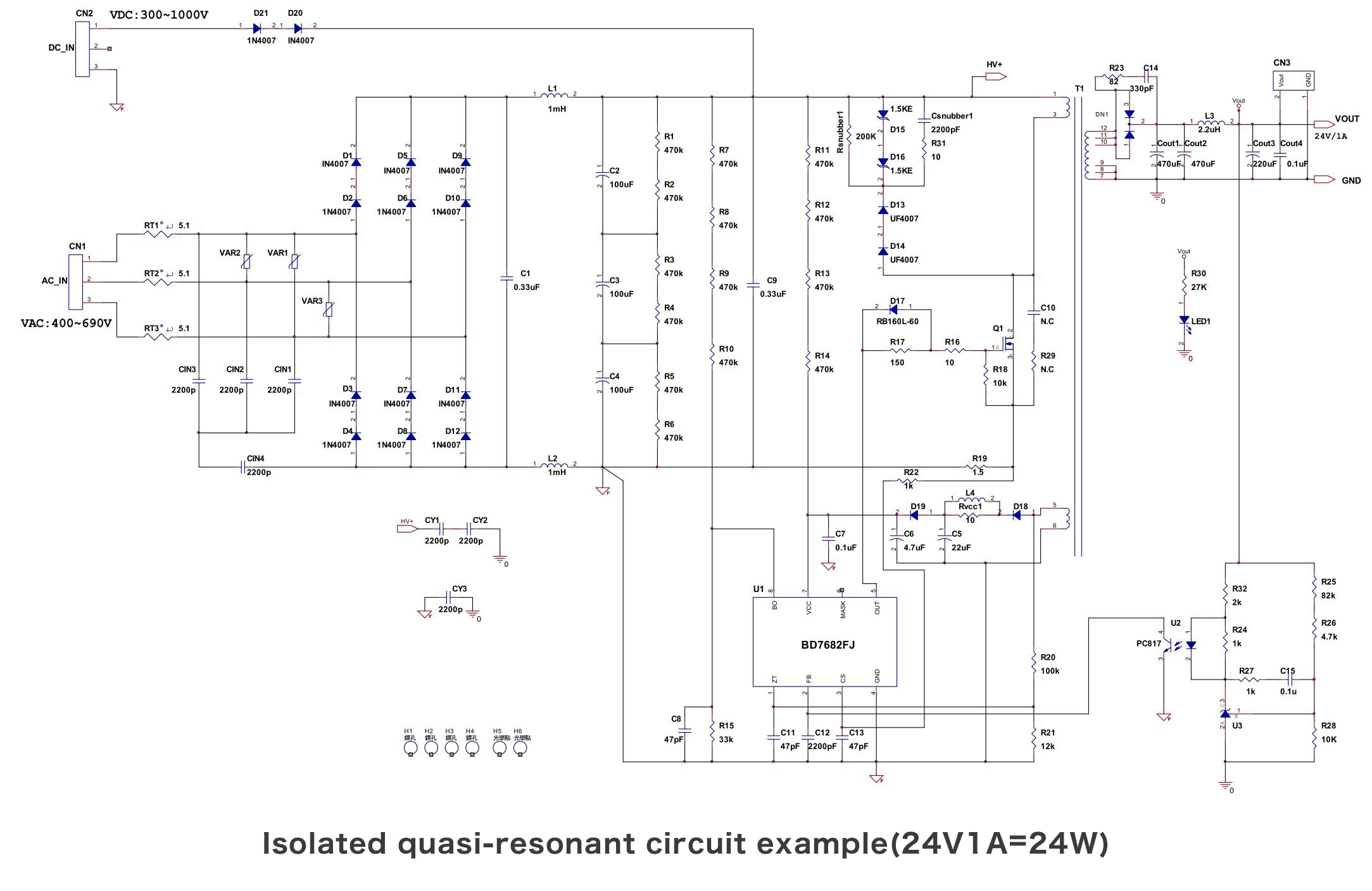# Design Example Circuit

2018.07.26

・A self-excitation type flyback converter using a quasi-resonant design utilizes the voltage resonance between the transformer primary winding inductor and a resonance capacitor.

・The quasi-resonant design makes possible reduced switching loss and noise levels.

In the previous section, we explained power supply ICs used in design. This time, we will describe a design example circuit.

## Quasi-Resonant Design

As explained the last time, the power supply IC used is the BD7682FJ-LB, an AC-DC converter controller IC used for SiC MOSFET driving. The conversion circuit uses a quasi-resonant design, in a self-excitation type flyback converter that uses the voltage resonance of the transformer primary winding inductor and a resonance capacitor. In general, such circuits make possible lower loss and noise levels compared with PWM flyback converters.

The circuit is in essence a flyback converter, and so energy accumulated in the transformer during MOSFET on-time is transmitted to the secondary side during MOSFET off-time. This operation is the same as in a PWM flyback converter, but in a quasi-resonant design, after the transformer has discharged energy, voltage oscillations occur due to resonance between the transformer primary winding inductor and the capacitance of a resonance capacitor. These voltage oscillations are used to cause the IC to detect the Vds bottom voltage and turn on the next cycle. When turn-on occurs with this timing, the current flowing in the transformer is zero and the drain voltage is also low, and so switching losses and noise can be reduced. These are advantages offered by the quasi-resonant design.It should be noted that switching losses in a quasi-resonant converter operating in this manner are dominated by losses during MOSFET off-time, and essentially no losses occur during MOSFET on-time.

Another feature of this operation is that under light loading, operation becomes discontinuous, and the switching frequency rises as the load is increased. Thereafter, boundary conduction mode operation occurs at a certain load current (boundary point), and in this state the switching frequency declines with increasing load. Thus the switching frequency changes with the load, and so this device can be called one kind of PFM converter.## Design Example of 24V/1A Isolated Quasi-Resonant Converter

The input/output conditions and circuit diagram for the design example are shown below. Based on these conditions, we calculate the values of the circuit components.

Output: 24V, 1A（24W）
Input: 300 to 900VDC（400 to 690VAC）

With respect to the input, both a DC voltage input and an AC voltage input are provided, but after rectification, the AC input voltage becomes a DC voltage, and so component values are set assuming a DC input voltage value.

## Click on the circuit diagram to enlarge it.